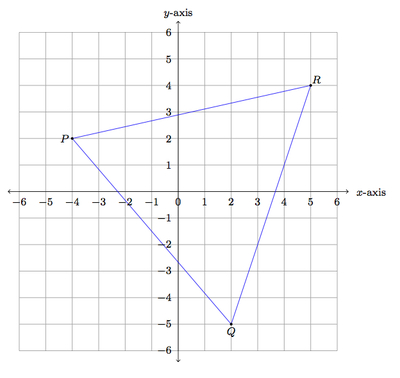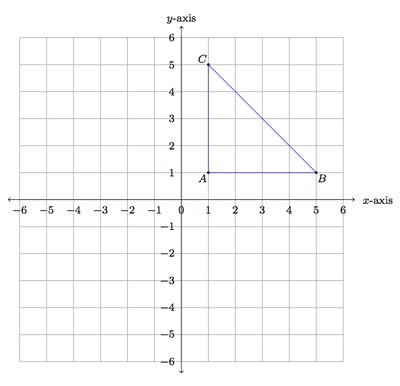# Finding isosceles triangles

Alignments to Content Standards: 8.G.B.8

Mrs. Lu has asked students in her class to find isosceles triangles whose vertices lie on a coordinate grid. For each student example below, explain why the triangle is isosceles.

1. Jessica draws the following triangle:2. Bruce's picture is here:3. Yan draws this picture:## IM Commentary

This task looks at some triangles in the coordinate plane and how to reason that these triangles are isosceles. One way to do this is to calculate side lengths using the Pythagorean Theorem. This method is not, however, always the most efficient. For the triangles given in parts (a) and (b) two of the legs are obtained by moving along the grid lines, from one vertex, by the same number of squares vertically and horizontally. Also, in parts (a) and (b), a line of reflective symmetry is not hard to identify. So in these two cases there are alternative explanations and the teacher may wish to emphasize this. For part (c), it is not easy to see that this triangle is isosceles without the Pythagorean Theorem. The teacher may wish to ask students to explain why the triangles in (a) and (b) are isosceles without using the Pythagorean Theorem if this does not come up in student work.

As an extension of (or introduction to) the activity presented here, the teacher may wish to prompt each student to draw an isosceles triangle whose vertices are on the coordinate grid points. They can then exchange examples and verify that the triangles are isosceles. If no student comes up with an example like the one in parts (b) and (c), the teacher can then introduce these. One type of isosceles triangle that students are likely to produce is a right isosceles triangle like the one below:In this case, $\overline{AC}$ and $\overline{AB}$ are both 4 units long: since they lie along the gridlines, this can be seen by counting boxes.

This task gives students a chance to engage in MP3, Construct Viable Arguments and Critique the Reasoning of Others. They need to explain why each of the given triangles is isosceles and different reasoning is likely to be applicable in the three cases. Moreover, if students are asked to create their own isosceles triangles and then exchange with one another, this provides an additional opportunity for them to analyze their classmates' reasoning.

This task is closely related to www.illustrativemathematics.org/illustrations/1245 where a rectangle is placed in the coordinate grid in a skew way. In order to do this, it is necessary to write a whole number as a sum of two squares in different ways. The number chosen there is 125 which can be written as

\begin{align} 125 &= 11^2 + 2^2 \\ &= 10^2 + 5^2. \end{align}

The relationship of this example with the current task is that it is possible to create an isosceles triangle by starting at a point, (0,0) for example, and then going right 11 and up 2 to (11,2) and then going right 10 and up 5 to (10,5). These three vertices make an isosceles triangle as we can see using the Pythagorean Theorem and the calculation above which shows that two of the sides have length $\sqrt{125}$.

## Solution

1. We can see that Jessica's triangle is isosceles in several ways. We will show that $|AC| = |BC|$. We can use the Pythagorean Theorem to check that these both have length $\sqrt{2^2 + 3^2}$. In the calculations below $M = (3,1)$ is the midpoint of $\overline{AB}$.

\begin{align} |AC|^2 &= |AM|^2 + |MC|^2 = 2^2 + 3^2\\ |BC|^2 &= |BM|^2 + |MC|^2 = 2^2 + 3^2. \end{align}

We can also observe that to get from $A$ to $C$ we go over (to the right) by two units and then up three units. To get from $B$ to $C$ we go over (to the left) by two units and then up three units. We travel the same distance to get from $A$ or $B$ to $C$ so $A$ and $B$ are equidistant from $C$. Finally, we can draw the horizontal line of symmetry for $\triangle ABC$:Isosceles triangles are exactly those triangles with a line of symmetry so $\triangle ABC$ must be isosceles.

2. The same three methods that applied to Jessica's triangle also apply to Bruce's triangle. We can use the Pythagorean Theorem to check that $\overline{DF}$ and $\overline{DE}$ both have length $\sqrt{4^2+1^2}$. In the calculations below $G = (5,1)$ and $H = (1,5)$: these points are labelled in the next picture which also shows the line of symmetry of Bruce's triangle.

\begin{align} |DF|^2 &= |DH|^2 + |HF|^2 = 4^2 + 1^2\\ |DE|^2 &= |DG|^2 + |GE|^2 = 4^2 + 1^2. \end{align}

We can also observe that to get from $D$ to $F$ we go over (to the right) by one unit and then up four units. To get from $D$ to $E$ we go over (to the right) by four units and then up one unit. We travel the same distance to get from $D$ to $F$ or $E$ so $F$ and $E$ are equidistant from $D$. Finally, we can draw the line of symmetry for $\triangle DEF$:To check that this is a line of symmetry, note that it is perpendicular to $\overleftrightarrow{EF}$ which we can see since it is one diagonal of the coordinate square where it meets $\overline{EF}$ with $\overline{EF}$ being the other diagonal. Also the lines meet at the midpoint of $\overline{EF}$, in the center of the coordinate square.

3. Yan's triangle is different from Jessica's and Bruce's triangles. If we count the number of boxes to get from one vertex to another (horizontally and vertically), we get different pairs of numbers for each pair of vertices. Also, the triangle does have a line of symmetry but it is not easy to identify this line from the picture. It is actually not even easy to distinguish which two sides have the same length.

So the best method for checking the side lengths of Yan's triangle is to use the Pythagorean Theorem. The coordinates for the three vertices are $P = (-4,2), Q = (2,-5), R = (5,4)$. With this information and the Pythagorean Theorem we can calculate the three side lengths:

\begin{align} |PQ|^2 &= 6^2 + 7^2 = 85 \\ |PR|^2 &= 9^2 +2^2 = 85 \\ |QR^2| &= 3^2 + 9^2 = 90. \end{align}

We can see that $|PQ| = |PR|$ and both are a little smaller than $|QR|$ but this difference is small enough that it is difficult to see in the picture. Since $|PQ| = |PR|$ we have shown that $\triangle PQR$ is isosceles.